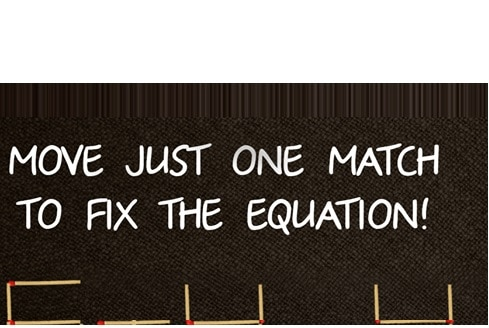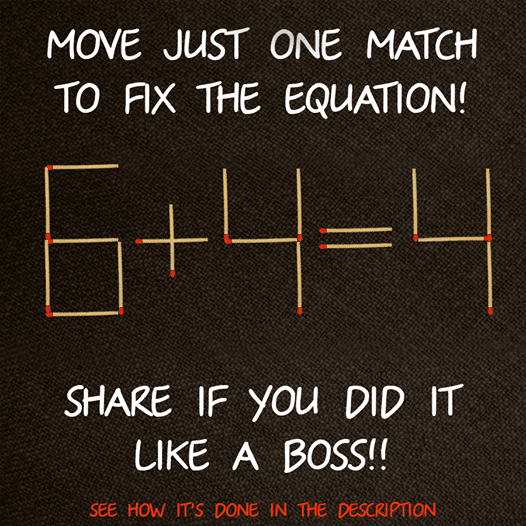Riddle: Move One Match to Make the Arithmetic Equation True - Examined Existence

# Riddle: Move One Match to Make the Arithmetic Equation TrueI think I’m going to start doing brain teasers here on a regular basis.  After all, this site is focused on the brain and how it works after all.  With this riddle, you must move only one match to make the math equation true.  Now, there are several answers, so think of as many right answers as possible and post in the comments section below.  Share with your friends to see how many they can get.By Tri
By Tri
By Tri
By Tri
By Tri
By Tri